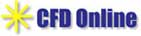Principles
2. Volumetric Correlation PIV (VCPIV)

Volumetric-correlation particle image velocimetry (VCPIV) is a new technique that provides a 3-dimensional 2-component velocity field from a single image plane. This single camera technique is simpler and cheaper to implement than multi-camera systems, and has the capacity to measure time varying flows. Additionally, this technique has significant advantages over other 3D PIV velocity measurement techniques most notably in the capacity to measure highly-seeded flows. Highly seeded flows, often unavoidable in industrial and biological flows, offer considerable advantages due to higher information density and better overall signal-to-noise ratio allowing for optimal spatial and temporal resolution.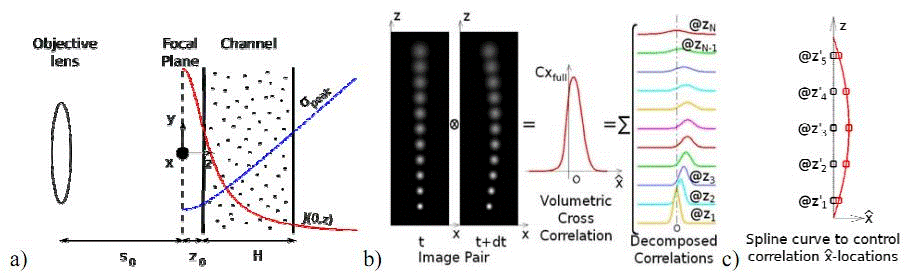Fig: Illustration of volumetric-correlation VPIV principle a) Schematic diagram including coordinate system for VPIV measurements (Fouras et al. ). The focal plane is at a working distance from the objective lens, and at distance from the channel to avoid the non-linear part of . The channel height H is chosen small enough to cover a region of strong correlation intensity for sufficient signal-to-noise ratio. The origin of the coordinates is at the focal plane. The principal flow direction is x-direction. b) The correlation of an image pair of particles distributed over the channel depth z yields a full volumetric-correlation peak. This peak can be considered as the sum of the correlations of all particles at different z locations. To obtain the velocity profile, the volumetric-correlation peak is decomposed into correlation peaks at (@) evenly-spaced z positions such that their sum equal to the given volumetric cross-correlation. c) Spline interpolation used to control horizontal locations of decomposed correlations at to to reduce the degree of freedom. The spline curve is the measured velocity profile when the optimization converges. Five nodes from to are used here, however more or less nodes are needed for more general velocity profiles.

the cross-correlation of an image pair containing particles distributed in the z direction is linearly proportional to the summation of the cross-correlations Cx2D (dx,dy, z ) of the particles at all z positions: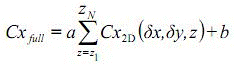where a and b are constants, and Cx2D (dx, dy, z) the cross-correlation function with the displacement components dx and dy of particles where z ranges from z1 to zN. The cross correlation Cx2D (dx,dy,z) can be expressed as a Gaussian function: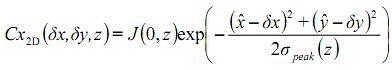Courtesy: Edited by: Mr. Bhupesh Doda, IIT Bombay# PIV Challenges# Resoures for Fluid dynamics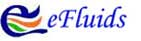# CFD Online Resoure